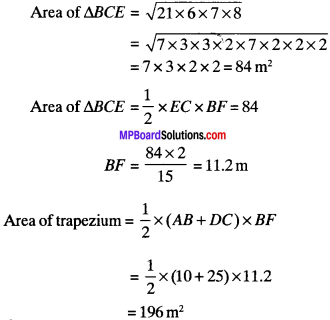## MP Board Class 9th Maths Solutions Chapter 12 Heron’s Formula Ex 12.2

Question 1.
A park, in the shape of a quadrilateral ABCD, has ∠C = 90°, AB = 9 m, BC = 12 m, CD, = 5 m and AD = 8 m. How much area does it. occupy?
Solution:
In ∆DCB
DB2 = DC2 + BC2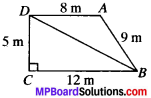In ∆DBA P = (8 + 9 + 13)m = 30m
s = $$\frac{P}{2}$$ = 15 m
s – a = 15 – 13 = 2
s – b = 15 – 9 = 6
s – c = 15 – 8 = 7
Area of ∆DBA = $$\sqrt{15x 2x6x7}$$
$$\sqrt{3x5x2x2x3x7}$$
= 2 x 3$$\sqrt{5×7}$$ x 7 = 6$$\sqrt{35}$$m2
Area of quadrilateral ABCD = (30 + 6$$\sqrt{35}$$) m2
= 30 + 6 x 5.91
= 30 + 35.46
= 65.46 m2Question 2.
Find the area of a quadrilateral ABCD in which AB = 3 cm, BC = 4 cm, CD = 4 cm, DA = 5 cm and AC = 5 cm.
Solution:
In ∆ABC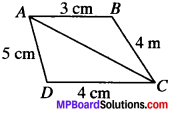P = 3 + 4 + 5 = 12cm
s = $$\frac{12}{2}$$ = 6 cm
s – a = 6 – 5 = 1 cm
s – b = 6 – 4 = 2cm
s – c = 6 – 3 = 3 cm
Area of ∆ABC = $$\sqrt{6x1x2x3}$$
= $$\sqrt{2x3x2x3}$$
= 2 x 3 = 6 cm2
In ∆ADC P = 5 + 5 + 4 = 14 cm
s = $$\frac{P}{2}$$ = 7 cm
s – a = 7 – 5 = 2cm
s – b = 7 – 5 = 2cm
s – c = 7 – 4 = 3cm
Area of ∆ADC = $$\sqrt{7x2x2x3}$$ = 2$$\sqrt{21}$$ cm2
Area of ∆BCD = Area of ∆ABC + Area of ∆ADC
= (6 + 2$$\sqrt{21}$$) cm2
= (6 + 2 x 4.58)
= 15.16 cm2

Question 3.
Radha made a picture of an aeroplane with coloured paper is shown in Fig. Find the total area of the paper used.
Solution:
The figure is divided into five parts as shown in Fig.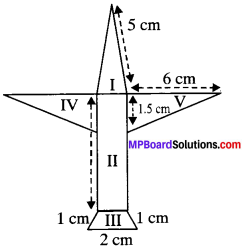• Part I – Triangle having sides 5 cm, 5 cm and 1 cm
• Part II – Rectangle having sides 1 cm and 6.5 cm
• Part III – Trapezium having sides 2,1,1,1.
• Part IV and V. Right angled triangles having sides 6 cm and 1.5 cm.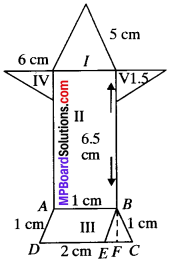For Part I:
a = 5 cm
b = 5 cm
c = 1 cm
s = $$\frac{P}{2}$$ = $$\frac{5+5+1}{2}$$ = $$\frac{11}{2}$$ = 5.5 cm
s – a = 5.5 – 5 = 0.5
s – b = 5.5 – 5 = 0.5
s – c = 5.5 – 1 = 4.5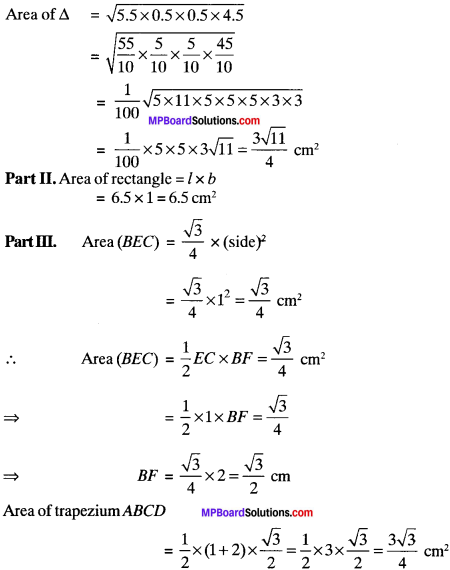Area of triangle IV and V = $$\frac{1}{2}$$ x 1.5 x 6 = 4.5 cm2
∴ Area of paper required = Area of part I + Area of part II + Area of part III + Area of part IV + Area of part V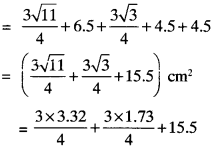= 2.49 + 1.27 + 15.5
= 19.26 cm2

Question 4.
A triangle and a parallelogram have the same base and the &me area. If the sides of the triangle are 26 cm, 28 cm and 30 cm, and the parallelogram stands on the base 28 cm, find the height of the parallelogram.
Solution: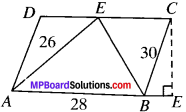In ∆ABE
a = 30 cm
b = 28 cm
c = 26 cm
s = $$\frac{P}{2}$$ = $$\frac{30+28+26}{2}$$ = 42 cm
s – a = 42 – 30 = 12
s – b = 42 – 28 = 14
s – c = 42 – 26 = 16
Area of ∆ABE = $$\sqrt{42x12x14x16}$$
= $$\sqrt{2x3x7x2x2x3x2x7x4x4}$$
= 2 x 3 x 7 x 2 x 4
= 336 cm2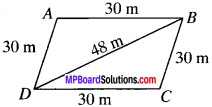Area of parallelogram ABCD = area of ∆ABE = 336 cm2 (given)
Area of parallelogram = b x h
336 = 28 x h
⇒ $$\frac{336}{28}$$
h= 12 cm.Question 5.
A rhombus shaped field has green grass for 18 cows to graze. If each side of the rhombus is 30 m and its longer diagonal is 48 m, how much area of grass field will each cow be getting?
Solution:
We know that the diagonal of a rhombus divide it into two triangles of equal area.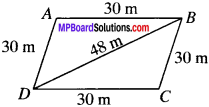a = 48m
b = 30m
c = 30m
s = $$\frac{P}{2}$$ = $$\frac{48+30+30}{2}$$ = $$\frac{108}{2}$$ = 54
s – a = 54 – 48 = 6 cm
s – b = 54 – 30 = 24 cm
s – c = 54 – 30 = 24 cm
Area of ∆ABD
= $$\sqrt{54x6x24x24}$$
= $$\sqrt{2x3x3x3x2x3x2x2x2x3x2x2x2x3}$$
= 2 x 3 x 3 x 2 x 2 x 3 x 2 = 432 cm2
Area of rhombus = 2 x 432 = 864 cm2
Area of grass field for each cow = $$\frac{864}{18}$$ = 48 cm2

Question 6.
An umbrella is made by stitching 10 triangular pieces of cloth of two different colours (see Fig.), each piece measurig 20 cm, 50 cm and 50 cm. How much cloth of each colour is required for the umberella?
Solution: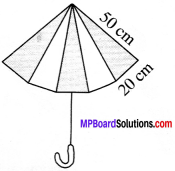a = 50 cm
b = 50cm
c = 20 cm
s = $$\frac{P}{2}$$ = $$\frac{50+50+20}{18}$$ = 60 cm
s – a = 60 – 50 = 10
s – b = 60 – 50 = 10
s – c = 60 – 20 = 40
Area of a ∆ = $$\sqrt{60x10x10x40}$$
= $$\sqrt{2x3x10x10x10x2x2x10}$$
= 10 x 10 x 52$$\sqrt{6}$$
= 200$$\sqrt{6}$$ cm2
Area of cloth of each type = 200$$\sqrt{6}$$ x 5 = 1000$$\sqrt{6}$$ cm2

Question 7.
A kite in the shape of a square with a diagonal 32 cm and an isosceles triangle of base 8 cm and sides 6 cm each is to be made of three different shades as shown in Fig. How much paper of each shade has been used in it?
Solution: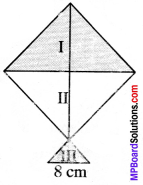∠AOB = 90°
BD = AC = 32 cm
OA = OC = 16 cm
Area (ABD) = area (DBC) = $$\frac{1}{2}$$ x 32 x 16 = 256 cm2
In ∆CEF
a = 6 cm
b = 6 cm
c = 8 cm
s = $$\frac{P}{2}$$ = $$\frac{6+6+8}{2}$$ = 10
s – a = 10 – 6 = 4
s – b = 10 – 6 = 4
s – c = 10 – 8 = 2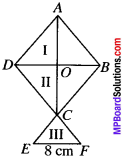Area of ∆CEF = $$\sqrt{10x4x4x2}$$
= $$\sqrt{5x2x4x4x2}$$
= 8$$\sqrt{5}$$ cm2
= 8 x 2.24 = 17.92 cm2Question 8.
A floral design on a floor is made up of 16 tiles which are triangular, the sides of the triangle being 9 cm, 28 cm and 35 cm (see Fig.). Find the cost of polishing the tiles at the rate of the field.
Solution: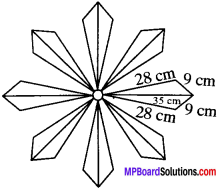a = 35 cm
b = 28 cm
c = 9 cm
s = $$\frac{P}{2}$$ = $$\frac{35+28+9}{2}$$
s = a = 36 – 35 = 1
5 = b = 36 – 28 = 8
c = c = 36 – 9 = 27
Area of a tile = $$\sqrt{36x1x8x27}$$
= $$\sqrt{2x2x3x3x2x2x2x3x3x3}$$
= 2 x 3 x 2 x 3$$\sqrt{6}$$ = 36$$\sqrt{6}$$ cm2
= 36 x 2.45 = 88.2 cm2
Total area of tiles = 16 x 88.2 = 1411.2 cm2
Cost of polishing the tiles per cm2 = 50 P
Total cost of polishing the tiles = 1411.2 x 50 P
= ₹ $$\frac{1411.2×50}{100}$$ = ₹ 705.60

Question 9.
A field is in the shape of a trapezium whose parallel sides are 25 m and 10 m. The non-parallel sides are 14 m and 13 m. Find the area of the field.
Solution:
ABCD is a trapezium. Draw BE parallel to AD. Draw BF ⊥ DC. ABED is a parallelogram
AB = DE = 10 m and AD = BE = 14 m
EC = DC – DE = 25 – 10 = 15 m
In ∆BEC
a = 15m
b = 14m
c = 13m
s = $$\frac{15+14+13}{2}$$ = 21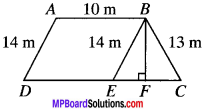s – a = 21 – 15 = 6
s – b = 21 – 14 = 7
s – c = 21 x 13 = 8